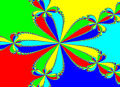# 2017APMO初選考試

 tsusy 寸絲發短消息 加為好友 當前離線 1# 大 中 小 發表於 2020-11-11 08:59  顯示全部帖子 推到噗浪 推到臉書 印象中是某年 APMO 初選，建議補上年份，方便後人查閱 設 $$c>a$$，則 $$0 < c - a < c$$，又 $$b \mid c -a$$，故 $$b < c$$ 因此 $$c$$ 是三質數之中最大者， 而 $$-2c < -2a < 7b - 2a < 7b < 7c$$，又 $$c \mid 7b - 2a$$，故 $$7b - 2a = -c, 0, c, 2c, 3c, 4c, 5c$$ 或 $$6c$$ 令 $$7b - 2a =kc$$，其中 $$-1 \leq k \leq 6$$ 且 $$k \in \mathbb Z$$ $$b \mid a-c \Rightarrow b \mid k(a-c)$$ 而 $$k(a-c) =(k+2)a - 7b$$，又 $$a,b,c$$ 為相異質數，故 $$b \mid k+2$$ $$a \mid 3b-c \Rightarrow b \mid k(3b-c)$$ 而 $$k(3b-c) =2a + (3k-7)b$$，又 $$a,b,c$$ 為相異質數，故 $$a \mid 3k-7$$ 把所有的 $$k = -1, 0, 1, \ldots, 6$$ 皆代入，即可找出 $$c>a$$ 條件下的所有解。 但題意有給 $$20 < c <80$$，可利用此條件快速判斷 $$b \mid k+2$$、 $$-1 \leq k \leq 6$$、$$b$$ 為質數，可得 $$b = 2, 3, 5, 7 \Rightarrow b\leq 7$$ 因此 $$kc = 7b-2a < 49 \Rightarrow k\leq 2$$ 僅需檢查 $$k = -1, 0, 1, 2$$，可得 $$k =0$$ 時，$$(a,b,c,) = (7,2,41)$$ 至於 \( c
﻿